# The Benefits Of Low Correlation: Round II

October 21, 2008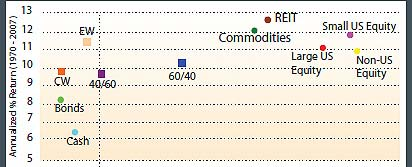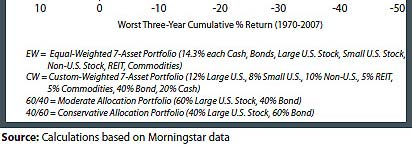Adding bonds to the cash portfolio (both equally weighted) improved the IRR to 7.71 percent but caused a slight increase in standard deviation (3.63 percent versus 3.08 percent in the all-cash portfolio). The aggregate correlation of the two-asset portfolio was 0.41. The worst-case one-year portfolio drawdown was dramatically reduced, from -13.9 percent to -3.4 percent. Moreover, the percentage of years with a loss was lowered from 53 percent to 24 percent, and the average annual loss was reduced from -4.4 percent to -2.0 percent. These improvements in the performance of the portfolio were not revealed by standard deviation-in fact, using only standard deviation of return as the measure of portfolio volatility would have completely missed the beneficial impact of adding a second asset to the portfolio.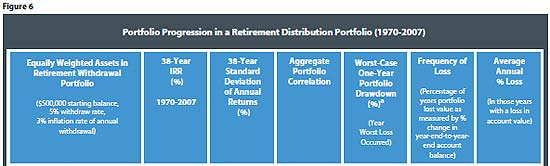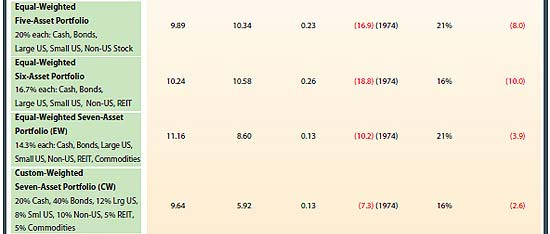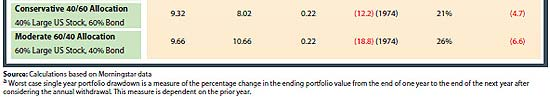Next, U.S. large-cap equities were added to the portfolio. The three assets (cash, bonds, large U.S. equities) were each equally weighted at 33.33 percent of the portfolio. With three assets, the aggregate correlation of the portfolio declined from 0.41 to 0.22. The 38-year IRR of the portfolio increased by 98 bps to 8.69 percent, but the standard deviation of return increased from 3.63 percent to 6.44 percent. The worst-case one-year portfolio drawdown increased to -9.4 percent. However, the three-asset portfolio only lost value 18 percent of the time, compared with 24 percent in the two-asset portfolio. Once again, standard deviation of return increases, but frequency of loss decreases as the aggregate portfolio correlation decreased.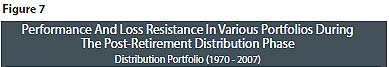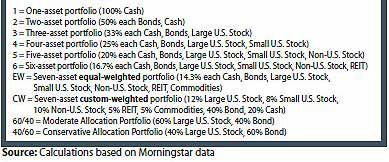When adding the fourth asset (small U.S. stocks), the aggregate portfolio correlation increased slightly to 0.25, portfolio return improved by 80 bps, standard deviation increased from 6.44 percent to 9.33 percent, worst one-year loss widened abruptly to -14.2 percent, frequency of loss increased slightly to 24 percent and the average loss increased from -4.3 percent to -5.3 percent. The inclusion of the fourth asset was beneficial to performance but detrimental to aggregate correlation, worst-case loss, frequency of loss and average loss. The reason for this is that small U.S. stocks have such a high correlation to large U.S. stocks (the prior asset added). Adding an asset that has a high correlation to an asset already in the portfolio is not nearly as helpful to the portfolio (in a holistic way) as adding an asset that has a low correlation. In this particular case, it lifted return but at the cost of greater loss exposure.

Adding non-U.S. stocks as the fifth asset (20 percent allocated to each asset) lifted performance by 40 bps while changing aggregate portfolio correlation very little. Standard deviation of return and worst one-year loss increased, as did the average one-year loss (to -8.0 percent from -5.3 percent in the four-asset portfolio). Same story as above, non-U.S. equity has fairly high correlation to U.S. equity.

The sixth asset added was REITs. The performance of the portfolio increased by 35 bps to 10.24 percent with standard deviation increasing only slightly. The aggregate portfolio correlation was 0.26 and the worst case one-year loss increased to -18.8 percent. Compared with the five-asset portfolio, the frequency of loss declined to 16 percent, but the average annual loss increased to -10.0 percent.

Commodities were added as the seventh asset, with each asset having a 14.3 percent allocation in the portfolio. Return increased by 92 bps to 11.16 percent and aggregate portfolio correlation dropped to 0.13. Standard deviation of return declined by nearly 200 bps and the worst case one-year loss was reduced from -18.8 percent to -10.2 percent. The portfolio's frequency of loss was 21 percent, and the average loss declined significantly to -3.9 percent. The impact of adding commodities is dramatic because it is an asset with low correlation to every other asset in the portfolio (refer back to Figure 2).

Also shown in Figure 6 are the results for a custom-weighted seven-asset portfolio (CW) which had a 20 percent allocation to cash, 40 percent to bonds, 12 percent to large U.S. equity, 8 percent to small U.S. equity, 10 percent to non-U.S. equity, 5 percent to REITs and 5 percent to commodities. The CW portfolio represents a conservative 40 percent equity/60 percent fixed income-with two 5 percent slices of the overall equity allocation being placed in low correlation "alternative"; assets (REITs and commodities). The CW portfolio is more likely to be implemented than the equally weighted seven-asset portfolio because a 28.6 percent allocation to REITs and commodities (in the equal-weighted portfolio) may be perceived as too risky.